#### The EM algorithm

The problem is often solved in practice by applying the expectation-maximization (EM) algorithm . In the general framework, there are three different spaces:

1. A set of parameters, which are to be determined through some measurement and estimation process. In our problem, this represents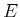, because the main goal is to determine the environment.
2. A set of data, which provide information that can be used to estimate the parameter. In the localization and mapping problem, this corresponds to the history I-space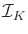. Each history I-state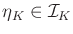is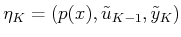, in which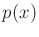is a prior probability density over.
3. A set of hidden variables, which are unknown but need to be estimated to complete the process of determining the parameters. In the localization and mapping problem, this is the configuration space.
Since both the parameters and the hidden variables are unknown, the choice between the two may seem arbitrary. It will turn out that expressions can be derived to nicely express the probability density for the hidden variables, but the parameters are much more complicated.

The EM algorithm involves an expectation step followed by a maximization step. The two steps are repeated as necessary until a solution with the desired accuracy is obtained. The method is guaranteed to converge under general conditions [269,977,978]. In practice, it appears to work well even under cases that are not theoretically guaranteed to converge .

From this point onward, let,, anddenote the three spaces for the EM algorithm because they pertain directly to the problem. Suppose that a robot has moved in the environment for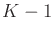stages, resulting in a final stage,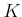. At each stage,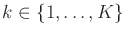, an observation,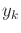, is made using its sensor. This could, for example, represent a set of distance measurements made by sonars or a range scanner. Furthermore, an action,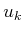, is applied for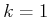to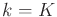. A prior probability density function,, is initially assumed over. This leads to the history I-state,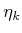, as defined in (11.14).

Now imagine thatstages have been executed, and the task is to estimate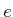. From each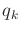, a measurement,, of part of the environment is taken. The EM algorithm generates a sequence of improved estimates of. In each execution of the two EM steps, a new estimate of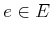is produced. Let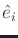denote this estimate after theth iteration. Let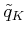denote the configuration history from stageto stage. The expectation step computes the expected likelihood of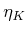given. This can be expressed as12.3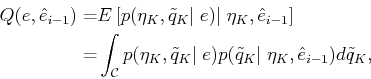(12.30)

in which the expectation is taken over the configuration histories. Sinceis given and the expectation removes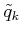, (12.30) is a function only ofand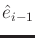. The term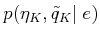can be expressed as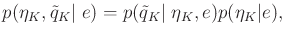(12.31)

in which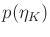is a prior density over the I-space, given nothing but the environment. The factor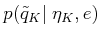differs from the second factor of the integrand in (12.30) only by usingor. The main difficulty in evaluating (12.30) is to evaluate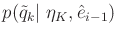(or the version that uses). This is essentially a localization problem with a given map, as considered in Section 12.2.3. The information up to stagecan be applied to yield the probabilistic I-state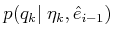for each; however, this neglects the information from the remaining stages. This new information can be used to make inferences about old configurations. For example, based on current measurements and memory of the actions that were applied, we have better information regarding the configuration several stages ago. In  a method of computingis given that computes two terms: One is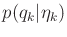, and the other is a backward probabilistic I-state that starts at stageand runs down to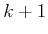.

Note that once determined, (12.30) is a function only ofand. The maximization step involves selecting anthat minimizes (12.30):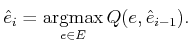(12.32)

This optimization is often too difficult, and convergence conditions exist ifis chosen such that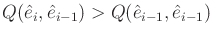. Repeated iterations of the EM algorithm result in a kind of gradient descent that arrives at a local minimum in.

One important factor in the success of the method is in the representation of. In the EM computations, one common approach is to use a set of landmarks, which were mentioned in Section 11.5.1. These are special places in the environment that can be identified by sensors, and if correctly classified, they dramatically improve localization. In , the landmarks are indicated by a user as the robot travels. Classification and positioning errors can both be modeled probabilistically and incorporated into the EM approach. Another idea that dramatically simplifies the representation ofis to approximate environments with a fine-resolution grid. Probabilities are associated with grid cells, which leads to a data structure called an occupancy grid [307,685,850]. In any case,must be carefully defined to ensure that reasonable prior distributions can be made for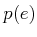to initialize the EM algorithm as the robot first moves.

Steven M LaValle 2020-08-14